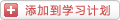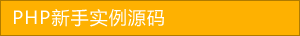﻿ 实现简单的php购物车代码 - 综合实例 - PHP粉丝网

# 实现简单的php购物车代码

##### 发布:smiling　来源: PHP粉丝网 　添加日期：2022-07-11 11:47:05　浏览: 评论：0

1. <body>
2.
3. <h1>登入页面</h1>
4.
5. <form action="dengrucl.php" method="post"
6.
7.   <p>帐号：<input type="text" name="zhang"/></p>
8.
9.   <p>密码：<input type="text" name="mi"/></p>
10.
11.   <input type="submit" value="登入"/>
12.
13. </form>
14.
15. </body>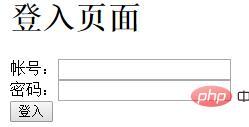1. <?php
2.
3. session_start();
4.
5. include ("db.class.php");
6.
7. \$db = new db();
8.
9. \$zhang = \$_POST["zhang"];
10.
11. \$mi = \$_POST["mi"];
12.
13. \$sql = "select mi from yonghu WHERE zhang = '{\$zhang}'"
14.
15. \$arr = \$db->Query(\$sql);
16.
17. if(!emptyempty(\$zhang)&&!emptyempty(\$mi)&&\$mi = \$arr){
18.
19.   \$_SESSION["zhang"] = \$zhang
20.
22.
23.
24. else
25.
26.
27.   echo "登入失败"
28.
29.
30.
31.
32. ?>

1. <body>
2.
3. <h1>长腿璇购物商城</h1>
4.
5. <table border="1" cellpadding="0" cellspacing="0" width="100%" >
6.
7.   <tr>
8.
9.     <td>代号</td>
10.
11.     <td>水果名称</td>
12.
13.     <td>水果价格</td>
14.
15.     <td>水果产地</td>
16.
17.     <td>水果库存</td>
18.
19.     <td>操作</td>
20.
21.   </tr>
22.
23.   <?php
24.
25.   session_start();
26.
27. \$_SESSION["zhang"] = "xiaoming";//不做登入的情况下，直接存sessiion
28.
29.   include ("db.class.php");
30.
31.   \$db = new db();
32.
33.   \$sql = "select * from sgbiao"
34.
35.   \$arr = \$db->Query(\$sql);
36.
37.   foreach (\$arr as \$v
38.
39.   {
40.
41.     echo " <tr>
42.
43.     <td>{\$v}</td>
44.
45.     <td>{\$v}</td>
46.
47.     <td>{\$v}</td>
48.
49.     <td>{\$v}</td>
50.
51.     <td>{\$v}</td>
52.
53.     <td>
54.
55.     <a href='zymcl.php?ids={\$v}'>加入购物车</a>
56.
57.
58.
59. </td>
60.
61.   </tr>";
62.
63.   }
64.
65.
66.
67.   ?>
68.
69.   <?php
70.
71.   //这里显示的是 购物车有多少产品，和产品的总价格
72.
73.   \$ann=array();
74.
75.   if(!emptyempty(\$_SESSION["gwc"]))
76.
77.   {
78.
79.     \$ann=\$_SESSION["gwc"];
80.
81.
82.
83.   }
84.
85.   \$zhonglei = count(\$ann);
86.
87.
88.
89.   \$aa=0;
90.
91.   foreach(\$ann as \$k
92.
93.   {
94.
95.
96.
97.     \$k;//水果代号
98.
99.     \$k;//水果数量
100.
101.     \$sql1="select jiage from sgbiao where ids='{\$k}'"
102.
103.
104.
105.     \$danjia=\$db->Query(\$sql1);
106.
107.
108.
109.     foreach(\$danjia as \$n
110.
111.     {
112.
113.
114.
115.       \$aa=\$aa + \$n*\$k;
116.
117.     }
118.
119.
120.
121.
122.
123.   }
124.
125.   echo"数量：{\$zhonglei}<br/>
126.
127. 价格:<mark>{\$aa}元";
128.
129.   ?>
130.
131. </table>
132.
133.
134.
135. <a href="tijiao.php" rel="external nofollow" rel="external nofollow" >查看账户</a>
136.
137. <a href="ckgwc.php" rel="external nofollow" >查看购物车</a>
138.
139.
140.
141. </body>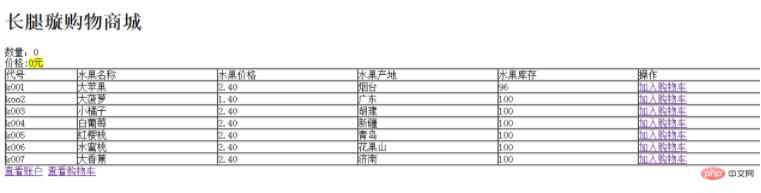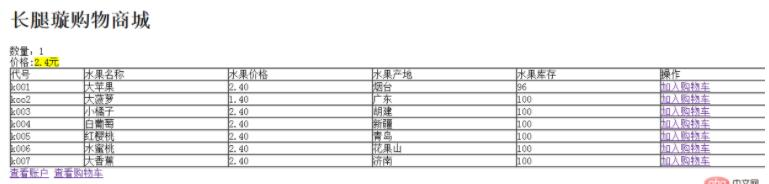1. <?php
2.
3. session_start();
4.
5. //
6.
7. \$ids = \$_GET["ids"];
8.
9. if(emptyempty(\$_SESSION["gwc"]))
10.
11.
12.   //如果点击的购物车是空的（第一次添加）
13.
14.
15.
16.   //如果购物车里是空的，造二维数组，
17.
18.   \$arr = array
19.
20.     array(\$ids,1)
21.
22.     //一维数组，取ids，第一次点击增加一个
23.
24.   );
25.
26.   \$_SESSION["gwc"]=\$arr
27.
28.   //扔到session里面
29.
30.
31. else
32.
33.   //这里不是第一次点击
34.
35.
36.   //先判断购物车里是否已经有了该商品，用\$ids
37.
38.   \$arr = \$_SESSION["gwc"];
39.
40.   //把购物车的状态取出来
41.
42.
43.
44.   \$chuxian = false;
45.
46. //定义一个变量；用来表示是否出现，默认是未出现
47.
48.   foreach (\$arr as \$v) {
49.
50.     //便利他
51.
52.     //如果这里面有这件商品
53.
54.     if (\$v == \$ids//如果取过来的\$v（商品的代号）等于\$ids那么就证明购物车中已经有了这一件商品
55.
56.     {
57.
58.       \$chuxian = true;
59.
60.       //如果出现，直接把chuxian改成true
61.
62.
63.
64.     }
65.
66.   }
67.
68.   if(\$chuxian
69.
70.   {
71.
72.     //购物车中有此商品
73.
74.     for(\$i=0;\$i<count(\$arr);\$i++)
75.
76.     {
77.
78.       if(\$arr[\$i] == \$ids
79.
80.       {
81.
82.         //把点到的商品编号加1
83.
84.         \$arr[\$i] += 1;
85.
86.       }
87.
88.     }
89.
90.     \$_SESSION["gwc"] = \$arr
91.
92.
93.
94.   }
95.
96.     else
97.
98.       {
99.
100.         //这里就只剩下：购物车里有东西，但是并没有这件商品
101.
102.         \$asg = array(\$ids,1);
103.
104.         //设一个小数组
105.
106.         \$arr[] = \$asg
107.
108.         \$_SESSION["gwc"]=\$arr
109.
110.       }
111.
112.
113.
114.
116.
117.
118.
119.
120.
121. ?>

1. <body>
2.
3. <h1>查看购物车</h1>
4.
5. <table width="100%" border="1"cellspacing="0" cellpadding="0"
6.
7.   <tr>
8.
9.     <td>商品名称</td>
10.
11.     <td>商品单价</td>
12.
13.     <td>商品数量</td>
14.
15.     <td>操作</td>
16.
17.   </tr>
18.
19.
20.
21.   <?php
22.
23.   session_start();
24.
25.   if(!emptyempty(\$_SESSION["gwc"]))
26.
27.   {
28.
29.     \$arr = array();
30.
31.     \$arr = \$_SESSION["gwc"];
32.
33.     //造数组
34.
35.   }
36.
37.   include ('db.class.php');
38.
39.   \$db = new db();
40.
41.   foreach (\$arr as \$v
42.
43.   {
44.
45.     global \$db
46.
47.     \$sql = "select * from sgbiao WHERE ids = '{\$v}'"
48.
49.     \$att = \$db->query(\$sql);
50.
51.     foreach (\$att as \$a
52.
53.     {
54.
55.       echo "<tr>
56.
57.     <td>{\$a}</td>
58.
59.     <td>{\$a}</td>
60.
61.     <td>{\$v}</td>
62.
63.     <td><a href='shanchu.php?ids={\$a}'>删除</a> </td>
64.
65.   </tr> ";
66.
67. //      蔬果的名称
68.
69. //      单价
70.
71. //      取int数量
72.
73. //    这个地方也可以加索引shanchu.php?sy={\$v}
74.
75.     }
76.
77.   }
78.
79.   ?>
80.
81.
82.
83. </table>
84.
85.
86.
87. <a href="tijiao.php" rel="external nofollow" rel="external nofollow" >提交订单</a>
88.
89. </body>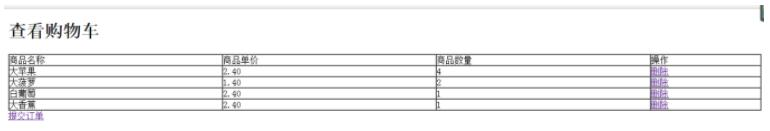1. <?php
2.
3. session_start();
4.
5. \$ids = \$_GET["ids"];
6.
7. \$arr = \$_SESSION["gwc"];
8.
9. //var_dump(\$arr);
10.
11. //取索引2（数量）
12.
13. foreach (\$arr as \$key=>\$v
14.
15.
16.   if(\$v==\$ids
17.
18.   {
19.
20.     if(\$v>1){
21.
22.       //要删除的数据
23.
24.       \$arr[\$key]-=1;
25.
26.     }
27.
28.     else
29.
30.       //数量为1的情况下，移除该数组
31.
32.       unset(\$arr[\$key]);
33.
34.     }
35.
36.   }
37.
38.
39.
40.
41.
42.
43. \$_SESSION["gwc"] = \$arr
44.
45. //记得扔到session里面
46.
48.
49. //删除完跳转回去

1. <?php
2.
3. session_start();
4.
5. include ("db.class.php");
6.
7. \$db = new db();
8.
9.
10.
11. //判断用余额是否满足
12.
13. \$zhang = \$_SESSION["zhang"];
14.
15. //获取到用户名
16.
17. \$sye = "select zhanghu from yonghu WHERE zhang = '{\$zhang}'"
18.
19. \$ye = \$db->query(\$sye);
20.
21. \$ye;//这是余额
22.
23.
24.
25. \$ann=array();
26.
27. if(!emptyempty(\$_SESSION["gwc"]))
28.
29.
30.   \$ann=\$_SESSION["gwc"];
31.
32.
33.
34.
35. \$zhonglei = count(\$ann);
36.
37.
38.
39. \$aa=0;//总价格
40.
41. foreach(\$ann as \$k
42.
43.
44.
45.
46.   \$k;//水果代号
47.
48.   \$k;//水果数量
49.
50.   \$sql1="select jiage from sgbiao where ids='{\$k}'"
51.
52.
53.
54.   \$danjia=\$db->Query(\$sql1);
55.
56.
57.
58.   foreach(\$danjia as \$n
59.
60.   {
61.
62.
63.
64.     \$aa=\$aa + \$n*\$k;
65.
66.   }
67.
68.
69.
70.
71.
72.
73. //判断余额是否满足
74.
75. if(\$ye>=\$aa
76.
77.
78.   //钱够,判断库存
79.
80.
81.
82.   foreach(\$ann as \$v
83.
84.   {
85.
86.     \$skc = "select sgname,kucun from sgbiao WHERE ids='{\$v}'"
87.
88.     //水果代号\$v
89.
90.     \$akc = \$db->query(\$skc);
91.
92.     \$akc;//库存
93.
94.     //比较是否满足库存
95.
96.     if(\$akc<\$v)
97.
98.     {
99.
100.       echo "{\$akc}库存不足"
101.
102.       //退出
103.
104.       exit
105.
106.     }
107.
108.
109.
110.   }
111.
112. //提交订单：
113.
114. //i.  从用户账户中扣除本次购买的总价格
115.
116. //ii.  从商品库存中扣除本次每种商品的购买数量
117.
118. //iii.  向订单表和订单内容表中加入本次购买的商品信息
119.
120.   //扣除账户余额
121.
122. \$skcye = "update yonghu set zhanghu = zhanghu-{\$aa} WHERE zhang = '{\$zhang}'"
123.
124.   \$db->query(\$skcye,0);
125.
126.   //扣除库存
127.
128.   foreach(\$ann as \$v
129.
130.   {
131.
132.     \$skckc = "update sgbiao set kucun = kucun-{\$v} WHERE ids='{\$v}'"
133.
134.     //水果代号\$v
135.
136.     \$db->query(\$skckc,0);
137.
138.   }
139.
140.   //添加订单信息
141.
142.   //取当前时间
143.
144.   \$time = time();
145.
146.   //自动生成订单号
147.
148.   \$ddh = date("YmdHis");
149.
150.   \$sdd = "insert into dingdan VALUES ('{\$ddh}','\$zhang','\$time')"
151.
152.   \$db->query(\$sdd,0);
153.
154.   //添加订单内容
155.
156.   foreach (\$ann as \$v
157.
158.   {
159.
160.     \$sddxq = "insert into ddneirong VALUES ('','\$ddh','{\$v}','{\$v}')"
161.
162.     \$db->query(\$sddxq,0);
163.
164.   }
165.
166.
167.
168.
169.
170.
171. else
172.
173.
174.   echo "钱不够"
175.
176.   exit
177.

1.减少水果库存：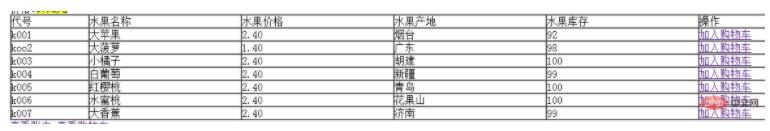2.添加订单：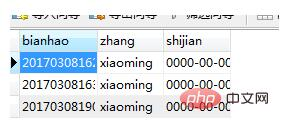3.添加订单内容：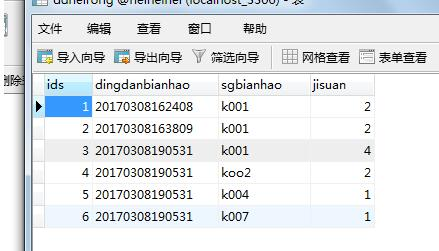4.扣除购买人的账户余额：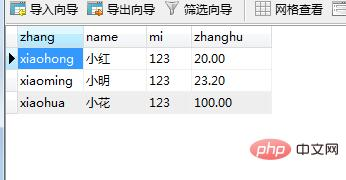### 分享到： document.write('<a href="http://v.t.sina.com.cn/share/share.php?url='+encodeURIComponent(location.href)+'&appkey=3172366919&title='+encodeURIComponent('实现简单的php购物车代码')+'" title="分享到新浪微博" class="t1" target="_blank">&nbsp;</a>'); document.write('<a href="http://www.douban.com/recommend/?url='+encodeURIComponent(location.href)+'&title='+encodeURIComponent('实现简单的php购物车代码')+'" title="分享到豆瓣" class="t2" target="_blank">&nbsp;</a>'); document.write('<a href="http://share.renren.com/share/buttonshare.do?link='+encodeURIComponent(location.href)+'&title='+encodeURIComponent('实现简单的php购物车代码')+'" title="分享到人人" class="t3" target="_blank">&nbsp;</a>'); document.write('<a href="http://www.kaixin001.com/repaste/share.php?rtitle='+encodeURIComponent('实现简单的php购物车代码')+'&rurl='+encodeURIComponent(location.href)+'&rcontent=" title="分享到开心网" class="t4" target="_blank">&nbsp;</a>'); document.write('<a href="http://sns.qzone.qq.com/cgi-bin/qzshare/cgi_qzshare_onekey?url='+encodeURIComponent(location.href)+'" title="分享到QQ空间" class="t5" target="_blank">&nbsp;</a>');